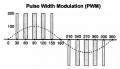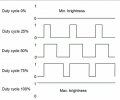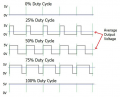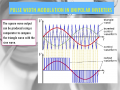# What exactly is the frequency of a PWM

#### rahulpsharma

Joined Sep 5, 2010
55
I have gained fruitful insights into PWM in other threads which are related to practical implementations for a specific ckt requirement...!!

However, while talking about PWM, I often keep getting confused about some theoretical aspects of the same...!! And so am posting a few of my 'confusions' here to seek answers from experts...!!

There few things to a PWM which I would like to know about:
1) Its own 'frequency' (I am not sure if I understand this one. Is there one...?? If there is one, what or how is it)
2) The freq of modulation: The frequency with which, the width of the pulse changes (This is understood fairly well but not in combination with 1) above)
3) Since, PWM has a 'freq', can a 1kHz pure sine wave, for instance, be compared with 1kHz PWM...?? I mean, freq of a sine wave, is loosely defined as the number of times the sine wave repeats itself every second...!! How would the corresponding definition for PWM would look like?
4) Since PWM is not a pure sinusoid, can a PWM be deconstructed as sums of sines and cosines of ever increasing frequencies...??

Rahul

#### Sensacell

Joined Jun 19, 2012
3,180
For any repeating signal, the frequency is = 1 / the time between rising edges.

#### Delta Prime

Joined Nov 15, 2019
1,311

#### Deleted member 115935

Joined Dec 31, 1969
0
PWM,
is a signal that has say a rising edge at a constant period apart, the falling edge is variable in time,
( can be reversed )

So the frequency is the "cycles per second" of the rising edge in this example,

To confuse, PDM, looks like PWM at first site,
but the period of rising edge , as well as the falling edge is normally variable.

( for a PWM, its down to the fourier of the series,
the fundamental sine wave of the series is the frequency of the PWM )

#### MrChips

Joined Oct 2, 2009
28,496
A sine wave (or sinusoidal wave) has one frequency, the fundamental frequency.
A sine wave also includes sine waves with a given phase angle offset, for example, cosine wave.

If the waveform is not sinusoidal then there are other frequencies in the mix, from a frequency spectrum perspective.
A delta function (a single pulse of infinitesimally short duration) has infinite frequencies.
Any waveform that has sharp rising or falling edges, e.g. square wave, will have infinite frequencies. Hence a PWM has very high frequencies, limited by the bandwidth of the source and transmission medium.
The fundamental frequency is the repetition rate of the PWM pulses.

#### Delta Prime

Joined Nov 15, 2019
1,311
Hello again!I mean, freq of a sine wave, is loosely defined as the number of times the sine wave repeats itself every second...!! How would the corresponding definition for PWM would look like?
For motor drive it would look like thisFor an LED you can vary the how bright you would like it.This is a correlation between duty cycle varying DC voltage .This next one is why you always here "pure sine wave"visual representation
Hopefully it will paint a picture in your mind of what other members accurately, have already stated.